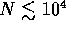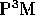Next: Small-N Systems Up: Orbits of Galaxies Previous: Trial Orbits

# N-body Algorithm

When studying the evolution of particle systems, whereas it be on atomic or cosmological scale, N-body simulations have proved to be a useful, and powerful, tool. If the particles can be considered as point masses, and preferably collisionless, such simulations have given very accurate results. The classical N-body problem simulates the evolution of a system of N bodies, where the force exerted on each body arises due to its interaction with all the other bodies in the system. N-body algorithms have numerous applications in areas such as molecular dynamics, plasma physics, and astrophysics. In astrophysics they have been used to study such diverse topics as planetary formation, star cluster dynamics, interacting galaxies, and even cosmological modeling.

In an N-body algorithm one calculates the force which acts on one particle from all the other particles in the system. The simulation proceeds over time-steps, each time computing the net force on every body, and thereby updating its position and other attributes. For a system where gravitation is the dominating force, the basic approach is that starting with the masses, positions and velocities of N particles at a given time, one can calculate, by numerically solving the equation of motion, the state of the system at any subsequent time. Doing this, one can get a good representation of the movements, the trajectories, of these particles. The main problem with this method, is that there is a limitation to the value of N due to computer time required to calculate the forces.

When having a relative small number of particles, i.e., direct summation is possible without making use of an impossible amount of CPU time. The method used is often known as ``particle-particle'' or PP scheme, where the force on a particle is calculated by directly summing the inverse square force law due to all other particles. Aboutoperations are required to evaluate the force on N particles from the other N-1 particles, and hence the computing time increases very rapidly with N. Nevertheless, the major cause for long computation time is the enormous difference in time scale from one particle to another, dependent on the distance between the particles. If particles gets close to each other, the velocities of the particles change very rapidly, whereupon correspondingly small time-steps is needed to follow their trajectories accurately. If there is no distinction between the time scales, the orbits of the more slowly varying particles will then be calculated at equally small, redundant, time-steps. A way to mend this problem is simply preventing the particles getting too close to each other by using a softening parameter. The strictforce law is then replaced by a smoothed version,, where c is the cutoff. Softening, and other methods, leads to a reduction of the computing time for the PP scheme, but not enough to make it usable for systems with large number of particles.

A significant gain in N can be obtained by calculating the force acting on a particle from a potential obtained by solving the Poisson equation on a mesh, instead of considering each particle individually. The ``particle-mesh'' or PM scheme adopts such an approach. There is an enormous reduction in computing time using this scheme opposed to the PP scheme, but it is at the cost of resolution in the evaluation of the force field. This scheme is therefore often used for systems in which the potential only varies at large scales, for example in HDM models.

A third scheme has been developed, namely the ``particle-particle-particle-mesh'' or thescheme. This method tries to combine the advantages of both the PP and PM methods. The basic idea is to split up the interparticle forces into two parts: a short range, rapidly varying, part due to nearby particles and a slowly varying part due to more widely separated particles. The PP method is used to determine the former and the PM scheme the latter; thus, one can evaluate the short range forces accurately and the long range forces rapidly. Due to the direct summations of the close range forces, thescheme is slower than the PM scheme, but faster than the PP scheme. Thehas been extensively used in cosmological simulations of CDM models.Next: Small-N Systems Up: Orbits of Galaxies Previous: Trial Orbits

Trond Hjorteland
Mon Jul 5 02:59:28 MET DST 1999